### Learn a new Times Table in only 5 days!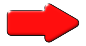Begin by writing out your times table on paper; slowly and carefully. Use addition to work out the next number in the sequence. Repeat this ten times then type out a neat copy to post onto the wall.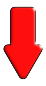### Fast Factors### Tables Conga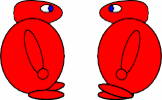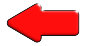### Tables Dash### Table Legs### Tables GrabMake up stories, jokes or rhymes to remind you of the difficult multiplication facts. Draw cartoon pictures to make them memorable. Post your work around the house or classroom.### Flash Tables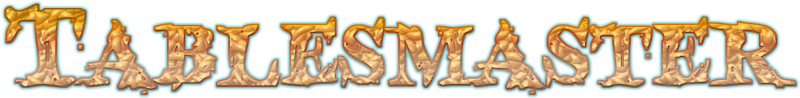The most popular activity on the Transum website!### Reverse Bar Chart### Beat The Clock### Playing Card Maths### Times Square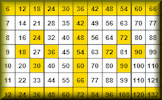### Expedite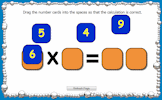### Connect 4 Factors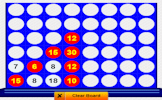### Convoluted### Hard   TimesTeachers: Start your lesson with a Times Tables Starter

Subscribers: Access our database of Times Tables Tips

### Times Tables

Learn a Times Table in only 5 days! Here are some free online activities (including Tablesmaster) to help you achieve your goal. There are games, exercises and speed tests designed to improve your recall of multiplication facts.

It is important to learn the times tables from 2 to 12. You should learn then as you'd learn a song or a dance. In fact you need to know your times tables forwards, backwards and all mixed up. Spend time learning them well and you'll reap the benefits in future.

Here are some printable forms on which you can record your progress: Personal Best Reverse Bar Chart | Tips For Remembering Multiplication Facts. Keep practising and one day you will be able to answer multiplication questions as fast as this:

The state of pupils' numeracy is always in the news and in particular politicians have pledged that every child in England will know their 12 times table before they leave primary school as part of a "war on innumeracy". They argue that there is still a case for learning your twelve times table, even though there are no longer 12 pence in a shilling, but the reason is to do with discovering patterns and building a confidence in handling numbers.

 1 x 1 = 12 x 1 = 23 x 1 = 34 x 1 = 45 x 1 = 56 x 1 = 67 x 1 = 78 x 1 = 89 x 1 = 910 x 1 = 1011 x 1 = 1112 x 1 = 12 1 x 2 = 22 x 2 = 43 x 2 = 64 x 2 = 85 x 2 = 106 x 2 = 127 x 2 = 148 x 2 = 169 x 2 = 1810 x 2 = 2011 x 2 = 2212 x 2 = 24 1 x 3 = 32 x 3 = 63 x 3 = 94 x 3 = 125 x 3 = 156 x 3 = 187 x 3 = 218 x 3 = 249 x 3 = 2710 x 3 = 3011 x 3 = 3312 x 3 = 36 1 x 4 = 42 x 4 = 83 x 4 = 124 x 4 = 165 x 4 = 206 x 4 = 247 x 4 = 288 x 4 = 329 x 4 = 3610 x 4 = 4011 x 4 = 4412 x 4 = 48 1 x 5 = 52 x 5 = 103 x 5 = 154 x 5 = 205 x 5 = 256 x 5 = 307 x 5 = 358 x 5 = 409 x 5 = 4510 x 5 = 5011 x 5 = 5512 x 5 = 60 1 x 6 = 62 x 6 = 123 x 6 = 184 x 6 = 245 x 6 = 306 x 6 = 367 x 6 = 428 x 6 = 489 x 6 = 5410 x 6 = 6011 x 6 = 6612 x 6 = 72 1 x 7 = 72 x 7 = 143 x 7 = 214 x 7 = 285 x 7 = 356 x 7 = 427 x 7 = 498 x 7 = 569 x 7 = 6310 x 7 = 7011 x 7 = 7712 x 7 = 84 1 x 8 = 82 x 8 = 163 x 8 = 244 x 8 = 325 x 8 = 406 x 8 = 487 x 8 = 568 x 8 = 649 x 8 = 7210 x 8 = 8011 x 8 = 8812 x 8 = 96 1 x 9 = 92 x 9 = 183 x 9 = 274 x 9 = 365 x 9 = 456 x 9 = 547 x 9 = 638 x 9 = 729 x 9 = 8110 x 9 = 9011 x 9 = 9912 x 9 = 108 1 x 10 = 102 x 10 = 203 x 10 = 304 x 10 = 405 x 10 = 506 x 10 = 607 x 10 = 708 x 10 = 809 x 10 = 9010 x 10 = 10011 x 10 = 11012 x 10 = 120 1 x 11 = 112 x 11 = 223 x 11 = 334 x 11 = 445 x 11 = 556 x 11 = 667 x 11 = 778 x 11 = 889 x 11 = 9910 x 11 = 11011 x 11 = 12112 x 11 = 132 1 x 12 = 122 x 12 = 243 x 12 = 364 x 12 = 485 x 12 = 606 x 12 = 727 x 12 = 848 x 12 = 969 x 12 = 10810 x 12 = 12011 x 12 = 13212 x 12 = 144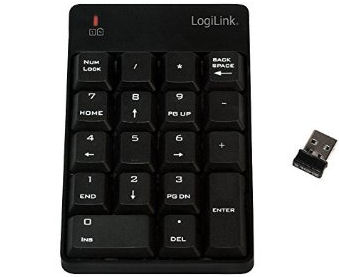Tablesmaster scores improve with a remote keypad

LogiLink Wireless Numeric Keypad### More Activities:

Transum,

Monday, November 3, 2014

"Another great idea for learning timestables is to make posters, each poster showing one of the more difficult tables facts.
Begin by doing the Tablesmaster activity. When you have finished you will find a list of the table facts and how long it took you to come up with the answer. Typically the longer times represent the facts that you had to work out rather than use instant recall. It is these facts that you should design a poster to help you remember. The posters should be funny or unusual so you can remember them and picture them when they are not in sight. The picture (use lots of colour) should relate directly to the multiplication fact and the answer.
Display your posters around the house so that you see them regularly. You will soon relate a tables fact with the room in the house where the poster is displayed and this will help you recall the table fact in the future."

Matt Curtis, Edgewood Primary School

Wednesday, March 9, 2016

"I love all of the times table resources you have created and the children in my class have really enjoyed using them. If you could create a high score leaderboard for the different games that was for each school, I think that would hugely drive up traffic to your site. I want to really promote your resources to my whole school and to parents at home and I think that if they could compete against other children they know, that would really inspire competition between them."

Transum,

Thursday, March 10, 2016

"That's a wonderful idea Matt, thanks for the suggestion. The easiest way to accomplish this is for you to ask your pupils to include the name of your school after their name when recording their score on the chart. For example if you have a pupil called John he could enter his name as John@Edgewood. You could then display a high-score on your whiteboard by entering Edgewood into the form on the newly created Customised High-Score page. Please shout out if you have any problems, questions or any other ideas. Thanks."

Wednesday, May 24, 2017

Saturday, January 27, 2018

Wednesday, February 14, 2018

Clive, Southampton

Tuesday, January 8, 2019

"I have been teaching for Maths for over 30 years and thought I knew my multiplication facts pretty well. I can quickly respond when any of the multiplication facts up to twelve times twelve and can easily spot common factors when factorising algebraic expressions. My grey cells were challenged however when trying to quickly sort the more tricky multiplication facts into order in the fastest possible time. You can try too by doing level 7 of the Snappy Sorting challenge. Here’s the link: Transum.org/Maths/Activity/Sort/?Level=7. Good Luck."